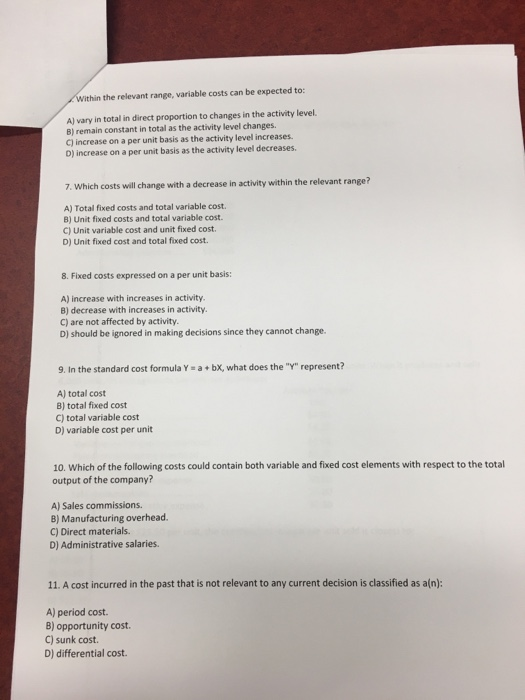# Question & Answer: Within the relevant range, variable costs can be expected to: A) vary in total in direct proportion to changes in the activ…..Within the relevant range, variable costs can be expected to: A) vary in total in direct proportion to changes in the activity level. B) remain constant in total as the activity level changes. C) increase on a per unit basis as the activity level increases. D) increase on a per unit basis as the activity level decreases. Which costs will change with a decrease in activity within the relevant range? A) Total fixed costs and total variable cost. B) Unit fixed costs and total variable cost. C) Unit variable cost and unit fixed cost. D) Unit fixed cost and total fixed cost. Fixed costs expressed on a per unit basis: A) increase with increases in activity. B) decrease with increases in activity. C) are not affected by activity. D) should be ignored in making decisions since they cannot change. In the standard cost formula Y = a + bx, what does the “Y” represent? A) total cost B) total fixed cost C) total variable cost D) variable cost per unit Which of the following costs could contain both variable and fixed cost elements with respect to the total output of the company? A) Sales commissions. B) Manufacturing overhead. C) Direct materials. D) Administrative salaries. A cost incurred in the past that is not relevant to any current decision is classified as a(n): A) period cost. B) opportunity cost. C) sunk cost. D) differential cost.

6) Variable costs are constant per unit and change in total while fixed costs always remain constant in total but change per unit within the relevant range
So, the answer is Option A

Don't use plagiarized sources. Get Your Custom Essay on
Question & Answer: Within the relevant range, variable costs can be expected to: A) vary in total in direct proportion to changes in the activ…..
GET AN ESSAY WRITTEN FOR YOU FROM AS LOW AS \$13/PAGE

7) We know that fixed cost per unit changes with the change in activity range as the fixed costs needs to be absorbed by the units produced. Variable costs always remain constant per unit and change as a total as they are dependant on the number of units produced,
So, the answer is Option B

8) As discussed above, the more the number of units produced the fixed cost per unit decreases per unit and the less the number of units produced, the more is the fixed cost per unit as the fixed costs are absorbed by lesser number of units due to lower production.
So, the answer is Option B

9) The formula for relationship of Total costs, Variable costs, Fixed costs are
Y= a+bx, where
Y = Total cost
a= Total fixed cost
b= Variable cost per unit
x= Number of units
So, the answer is Option A

10) We know that sales commission, Direct material costs are linked to production and are always variable. Administartive salaries are always fixed in nature and are not linked to production. Whereas manufacturing overheads contain fixed manufacturing costs and variable manufacturing costs of production.
So, the answer is Option B

11) Costs that are incurred in the past and are not relevant in decision making are called HISTORICAL costs or SUNK costs.
So, the answer is Option C